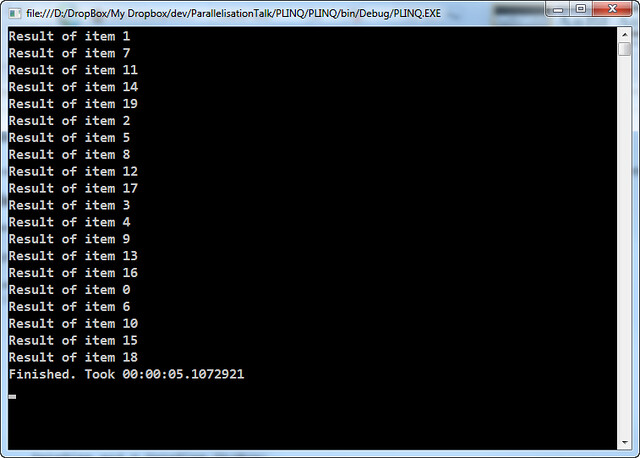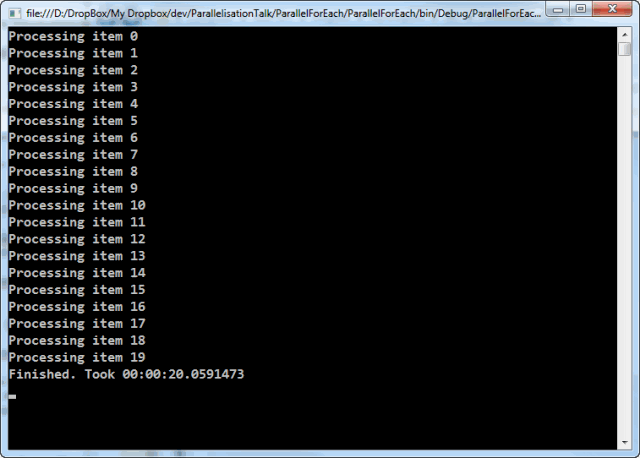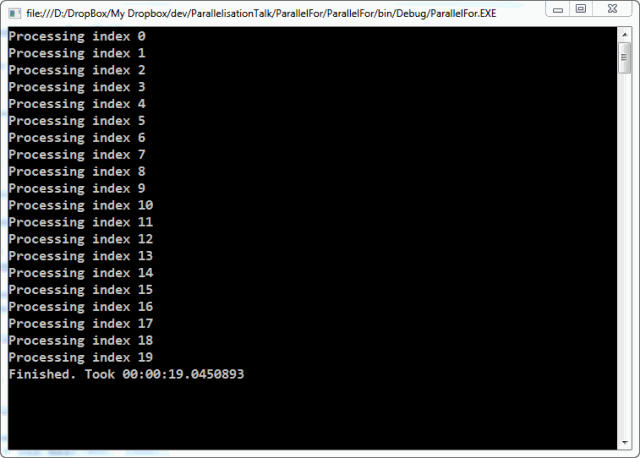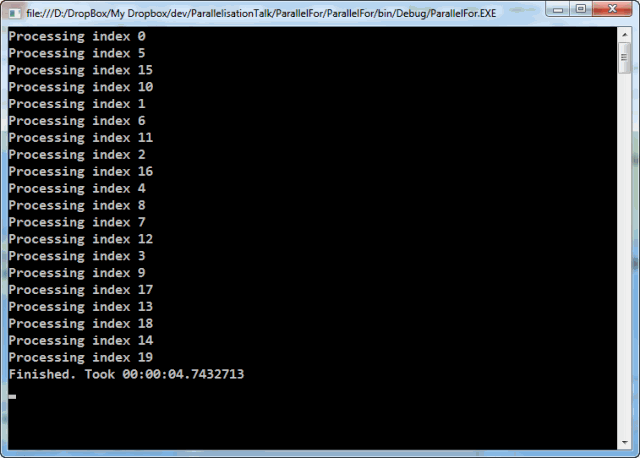## Parallelisation Talk Example – ConcurrentBag

This example shows a `ConcurrentBag` being populated and it being accessed while another task is still populating the bag.

The `ConcurrentBag` class can be found in the `System.Collections.Concurrent` namespace

In this example, the `ConcurrentBag` is populated in task that is running in the background. After a brief pause in order to allow the background task time to put some items in the bag, main thread starts outputting the contents of the bag.

When the code starts to iterate over the bag, a snapshot is taken so that the enumeration is not tripped up by additional items being added or removed from the bag elsewhere. You can see this effect as only 13 items are output, yet immediately afterwards the bag has 20 items (in this example, if you run the code yourself you may get different results)

### Code Example

```class Program
{
private static ConcurrentBag<string> bag = new ConcurrentBag<string>();
static void Main(string[] args)
{
// Start a task to run in the background.

// Wait a wee bit so that the bag can get populated
// with some items before we attempt to output them.

// Display the contents of the bag
int count = 0;
foreach (string item in bag)
{
count++;
Console.WriteLine(item);
}

// Show the difference between the count of items
// displayed and the current state of the bag
Console.WriteLine("{0} items were output", count);
Console.WriteLine("The bag contains {0} items", bag.Count);

}

public static void PopulateBag()
{
for (int i = 0; i < 200; i++ )
{

// Wait a bit to simulate other processing.
}

// Show the final size of the bag.
Console.WriteLine("Finished populating the bag with {0} items.", bag.Count);
}
}```

### Typical output```This is item 12
This is item 11
This is item 10
This is item 9
This is item 8
This is item 7
This is item 6
This is item 5
This is item 4
This is item 3
This is item 2
This is item 1
This is item 0
13 items were output
The bag contains 20 items
Finished populating the bag with 200 items.```

## Parallelisation Talk Examples – ConcurrentDictionary

The example used in the talk was one I had already blogged about. The original blog entry the example was based upon is here: Parallelisation in .NET 4.0 – The ConcurrentDictionary.

### Code Example

```class Program
{
private static ConcurrentDictionary<string, int> wordCounts =
new ConcurrentDictionary<string, int>();

static void Main(string[] args)
{
Parallel.ForEach(lines, ProcessLine);

Console.WriteLine("There are {0} distinct words", wordCounts.Count);
var topForty = wordCounts.OrderByDescending(kvp => kvp.Value).Take(40);
foreach (KeyValuePair word in topForty)
{
Console.WriteLine("{0}: {1}", word.Key, word.Value);
}
}

private static void ProcessLine(string line)
{
var words = line.Split(' ')
.Select(w => w.Trim().ToLowerInvariant())
.Where(w => !string.IsNullOrEmpty(w));
foreach (string word in words)
CountWord(word);
}

private static void CountWord(string word)
{
UpdateCount(word);
}

private static void UpdateCount(string word)
{
int value = wordCounts[word];
if (!wordCounts.TryUpdate(word, value + 1, value))
{
Console.WriteLine("Failed to count '{0}' (was {1}), trying again...",
word, value);

UpdateCount(word);
}
}
}```

## Parallelisation Talk Examples – Basic PLINQ

These are some code examples from my introductory talk on Parallelisation showing the difference between a standard sequential LINQ query and its parallel equivalent.

The main differences between this and the previous two examples (Parallel.For and Parallel.ForEach) is that LINQ (and PLINQ) is designed to return data back, so the LINQ expression uses a `Func<TResult, T1, T2, T3…>` instead of an `Action<T1, T2, T3…>`. Since the examples were simply outputting a string to the Console to indicate which item or index was being processed I’ve changed the code to return a string back to the LINQ expression. The results are then looped over and output to the console.

It is also important to remember that LINQ expressions are not evaluated until the data is called for. In the example below that is with the `.ToList()` method call, however it may also be as a result of `foreach` or any other method of iterating over the expression results.

## Code example 1: Sequential processing of data with LINQ

```class Program
{
private static Random rnd = new Random();

static void Main(string[] args)
{
DateTime start = DateTime.UtcNow;

IEnumerable<int> items = Enumerable.Range(0, 20);

var results = items
.Select(ProcessItem)
.ToList();

results.ForEach(Console.WriteLine);

DateTime end = DateTime.UtcNow;
TimeSpan duration = end - start;

Console.WriteLine("Finished. Took {0}", duration);

}

private static string ProcessItem(int item)
{
// Simulate similar but slightly variable length processing
int pause = rnd.Next(900, 1100);

return string.Format("Result of item {0}", item);
}
}```

The output of the above code may look something like this:As you can see this takes roughly of 20 seconds to process 20 items with each item taking about one second to process.

## Code Example 2: Parallel processing of data with PLINQ

The `AsParallel` extension method can be found in the `System.Linq` namespace so no additional using statements are needed if you are already using LINQ.

```class Program
{
private static Random rnd = new Random();

static void Main(string[] args)
{
DateTime start = DateTime.UtcNow;

IEnumerable<int> items = Enumerable.Range(0, 20);

var results = items.AsParallel()
.Select(ProcessItem)
.ToList();

results.ForEach(Console.WriteLine);

DateTime end = DateTime.UtcNow;
TimeSpan duration = end - start;

Console.WriteLine("Finished. Took {0}", duration);

}

private static string ProcessItem(int item)
{
// Simulate similar but slightly variable length processing
int pause = rnd.Next(900, 1100);

return string.Format("Result of item {0}", item);
}
}```

The output of the above code may look something like this:The result of this code is that it takes roughly 5 second to process the 20 items. I have a 4 core processor so it would be in line with the expectation that the work is distributed across all 4 cores.

## Parallelisation Talk Examples – Parallel.ForEach

These are some code examples from my introductory talk on Parallelisation. Showing the difference between a standard sequential foreach loop and its parallel equivalent.

## Code example 1: Serial processing of a foreach loop

```class Program
{
private static Random rnd = new Random();

static void Main(string[] args)
{
DateTime start = DateTime.UtcNow;

IEnumerable items = Enumerable.Range(0,20);

foreach(int item in items)
ProcessLoop(item);

DateTime end = DateTime.UtcNow;
TimeSpan duration = end - start;

Console.WriteLine("Finished. Took {0}", duration);

}

private static void ProcessLoop(int item)
{
Console.WriteLine("Processing item {0}", item);

// Simulate similar but slightly variable length processing
int pause = rnd.Next(900, 1100);
}
}```

The output of the above code may look something like this:As you can see this takes roughly of 20 seconds to process 20 items with each item taking about one second to process.

## Code Example 2: Parallel processing of a foreach loop

The `Parallel` class can be found in the `System.Threading.Tasks` namespace.

```class Program
{
private static Random rnd = new Random();

static void Main(string[] args)
{
DateTime start = DateTime.UtcNow;

IEnumerable items = Enumerable.Range(0,20);

Parallel.ForEach(items,
(item) => ProcessLoop(item));

DateTime end = DateTime.UtcNow;
TimeSpan duration = end - start;

Console.WriteLine("Finished. Took {0}", duration);

}

private static void ProcessLoop(int item)
{
Console.WriteLine("Processing item {0}", item);

// Simulate similar but slightly variable length processing
int pause = rnd.Next(900, 1100);
}
}```

The output of the above code may look something like this:The result of this code is that it takes roughly 5 second to process the 20 items. I have a 4 core processor so it would be in line with the expectation that the work is distributed across all 4 cores.

## Parallelisation Talk examples – Parallel.For

This is some example code from my introductory talk on Parallelisation. Showing the difference between a standard sequential for loop and its parallel equivalent.

## Code example 1: Serial processing of a for loop

```class Program
{
private static Random rnd = new Random();

static void Main(string[] args)
{
DateTime start = DateTime.UtcNow;

for (int i = 0; i < 20; i++)
ProcessLoop(i);

DateTime end = DateTime.UtcNow;
TimeSpan duration = end - start;

Console.WriteLine("Finished. Took {0}", duration);
}

private static void ProcessLoop(long i)
{
Console.WriteLine("Processing index {0}", i);

// Simulate similar but slightly variable length processing
int pause = rnd.Next(900, 1000);
}
}```

The output of the above code may look something like this:As you can see this takes just shy of 20 seconds to process 20 items.

## Code Example 2: Parallel processing of a for loop

The `Parallel` class can be found in the `System.Threading.Tasks` namespace.

```class Program
{
private static Random rnd = new Random();

static void Main(string[] args)
{
DateTime start = DateTime.UtcNow;

Parallel.For(0, 20,
(i) => ProcessLoop(i));

DateTime end = DateTime.UtcNow;
TimeSpan duration = end - start;

Console.WriteLine("Finished. Took {0}", duration);

}

private static void ProcessLoop(long i)
{
Console.WriteLine("Processing index {0}", i);

// Simulate similar but slightly variable length processing
int pause = rnd.Next(900, 1000);